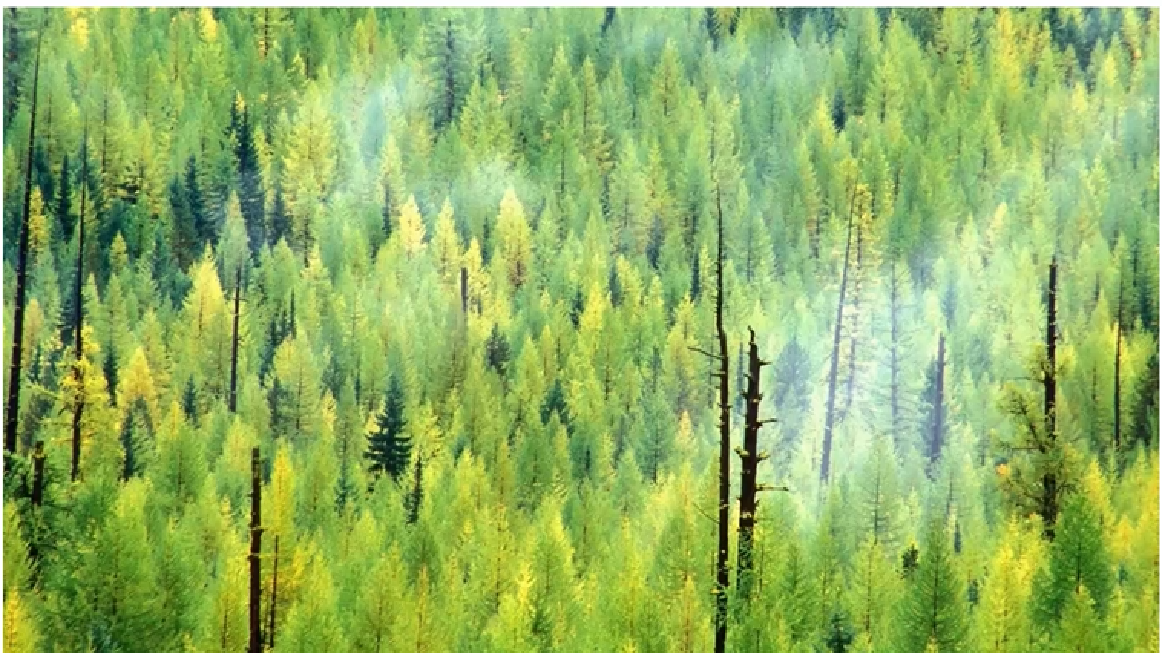# What is Random Forest Regression?

Original article was published by Manik Soni on Artificial Intelligence on Medium# What is Random Forest Regression?

## sklearn.ensemble.RandomForestRegressor

Before we dive deep into Random Forest Regression we first analyze what is a decision tree? and how the algorithm works?

Prerequisite: What is Decision Tree Regression?

Random forest Regression is a Non-linear machine learning model. Just like decision tree regression helps us to do predictions on a particular split, but in decision tree regression only one tree helps to do a prediction. In random forest ’N’ number of trees is doing prediction and the average of all the results of the tree helps to give accurate results.

Averaging helps to improve predictive accuracy and control over-fitting.

Random forest Regression is a type of ensemble learning technique where we take an average of all the results.

Steps to follow Random Forest Regression:

Step1. Pick at random ‘K’ data points from the Training set.

Step 2. Build the Decision Tree associated with these K data points.

Step 3. Choose the number ‘N tree’ of trees you want to build and repeat Steps 1&2.

Step 4. For new data points, make each one of your ‘N tree’ trees predict the value of Y to for the data point in question and assign the new data point the average across all of the predicted Y values.

Now we will do the practical implementation of the Random Forest Regression , we are importing the dataset of people having a position salary.

Now follow the steps to do Prediction:

Step 1: Import the Libraries

Step 2 : Importing the Dataset

Step 3: Split the data into a matrix of features(X) and the dependent variable(y).

Step 4: Fitting a linear model to test and training dataset.

Step 5: Predicting the Test result.

Step 6: Visualization of the dataset.